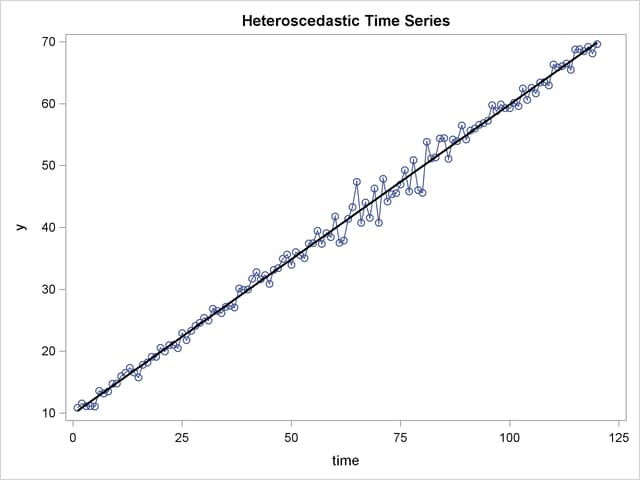The AUTOREG Procedure
 Testing for Heteroscedasticity

One of the key assumptions of the ordinary regression model is that the errors have the same variance throughout the sample. This is also called the homoscedasticity model. If the error variance is not constant, the data are said to be heteroscedastic.

Since ordinary least squares regression assumes constant error variance, heteroscedasticity causes the OLS estimates to be inefficient. Models that take into account the changing variance can make more efficient use of the data. Also, heteroscedasticity can make the OLS forecast error variance inaccurate because the predicted forecast variance is based on the average variance instead of on the variability at the end of the series.

To illustrate heteroscedastic time series, the following statements create the simulated series Y. The variable Y has an error variance that changes from 1 to 4 in the middle part of the series.

```data a;
do time = -10 to 120;
s = 1 + (time >= 60 & time < 90);
u = s*rannor(12346);
y = 10 + .5 * time + u;
if time > 0 then output;
end;
run;

title 'Heteroscedastic Time Series';
proc sgplot data=a noautolegend;
series x=time y=y / markers;
reg x=time y=y / lineattrs=(color=black);
run;
```

The simulated series is plotted in Figure 8.10.

Figure 8.10 Heteroscedastic and Autocorrelated SeriesTo test for heteroscedasticity with PROC AUTOREG, specify the ARCHTEST option. The following statements regress Y on TIME and use the ARCHTEST= option to test for heteroscedastic OLS residuals:

```/*-- test for heteroscedastic OLS residuals --*/
proc autoreg data=a;
model y = time / archtest;
output out=r r=yresid;
run;
```

The PROC AUTOREG output is shown in Figure 8.11. The Q statistics test for changes in variance across time by using lag windows that range from 1 through 12. (See the section Testing for Nonlinear Dependence: Heteroscedasticity Tests for details.) The p-values for the test statistics strongly indicate heteroscedasticity, with p < 0.0001 for all lag windows.

The Lagrange multiplier (LM) tests also indicate heteroscedasticity. These tests can also help determine the order of the ARCH model that is appropriate for modeling the heteroscedasticity, assuming that the changing variance follows an autoregressive conditional heteroscedasticity model.

Figure 8.11 Heteroscedasticity Tests
 Heteroscedastic Time Series

The AUTOREG Procedure

Dependent Variable y

Ordinary Least Squares Estimates
SSE 223.645647 DFE 118
MSE 1.89530 Root MSE 1.37670
SBC 424.828766 AIC 419.253783
MAE 0.97683599 AICC 419.356347
MAPE 2.73888672 HQC 421.517809
Durbin-Watson 2.4444 Regress R-Square 0.9938
Total R-Square 0.9938

Tests for ARCH Disturbances Based on OLS Residuals
Order Q Pr > Q LM Pr > LM
1 19.4549 <.0001 19.1493 <.0001
2 21.3563 <.0001 19.3057 <.0001
3 28.7738 <.0001 25.7313 <.0001
4 38.1132 <.0001 26.9664 <.0001
5 52.3745 <.0001 32.5714 <.0001
6 54.4968 <.0001 34.2375 <.0001
7 55.3127 <.0001 34.4726 <.0001
8 58.3809 <.0001 34.4850 <.0001
9 68.3075 <.0001 38.7244 <.0001
10 73.2949 <.0001 38.9814 <.0001
11 74.9273 <.0001 39.9395 <.0001
12 76.0254 <.0001 40.8144 <.0001

Parameter Estimates
Variable DF Estimate Standard Error t Value Approx
Pr > |t|
Intercept 1 9.8684 0.2529 39.02 <.0001
time 1 0.5000 0.003628 137.82 <.0001

The tests of Lee and King (1993) and Wong and Li (1995) can also be applied to check the absence of ARCH effects. The following example shows that Wong and Li’s test is robust to detect the presence of ARCH effects with the existence of outliers.

```/*-- data with outliers at obervation 10 --*/
data b;
do time = -10 to 120;
s = 1 + (time >= 60 & time < 90);
u = s*rannor(12346);
y = 10 + .5 * time + u;
if time = 10 then
do; y = 200; end;
if time > 0 then output;
end;
run;
/*-- test for heteroscedastic OLS residuals --*/
proc autoreg data=b;
model y = time / archtest=(qlm) ;
model y = time / archtest=(lk,wl) ;
run;
```

As shown in Figure 8.12, the p-values of Q or LM statistics for all lag windows are above 90%, which fails to reject the null hypothesis of the absence of ARCH effects. Lee and King’s test, which rejects the null hypothesis for lags more than 8 at 10% significance level, works better. Wong and Li’s test works best, rejecting the null hypothesis and detecting the presence of ARCH effects for all lag windows.

Figure 8.12 Heteroscedasticity Tests
 Heteroscedastic Time Series

The AUTOREG Procedure

Tests for ARCH Disturbances Based on OLS Residuals
Order Q Pr > Q LM Pr > LM
1 0.0076 0.9304 0.0073 0.9319
2 0.0150 0.9925 0.0143 0.9929
3 0.0229 0.9991 0.0217 0.9992
4 0.0308 0.9999 0.0290 0.9999
5 0.0367 1.0000 0.0345 1.0000
6 0.0442 1.0000 0.0413 1.0000
7 0.0522 1.0000 0.0485 1.0000
8 0.0612 1.0000 0.0565 1.0000
9 0.0701 1.0000 0.0643 1.0000
10 0.0701 1.0000 0.0742 1.0000
11 0.0701 1.0000 0.0838 1.0000
12 0.0702 1.0000 0.0939 1.0000

Tests for ARCH Disturbances Based on OLS Residuals
Order LK Pr > |LK| WL Pr > WL
1 -0.6377 0.5236 34.9984 <.0001
2 -0.8926 0.3721 72.9542 <.0001
3 -1.0979 0.2723 104.0322 <.0001
4 -1.2705 0.2039 139.9328 <.0001
5 -1.3824 0.1668 176.9830 <.0001
6 -1.5125 0.1304 200.3388 <.0001
7 -1.6385 0.1013 238.4844 <.0001
8 -1.7695 0.0768 267.8882 <.0001
9 -1.8881 0.0590 304.5706 <.0001
10 -2.2349 0.0254 326.3658 <.0001
11 -2.2380 0.0252 348.8036 <.0001
12 -2.2442 0.0248 371.9596 <.0001Previous Page | Next Page | Top of Page